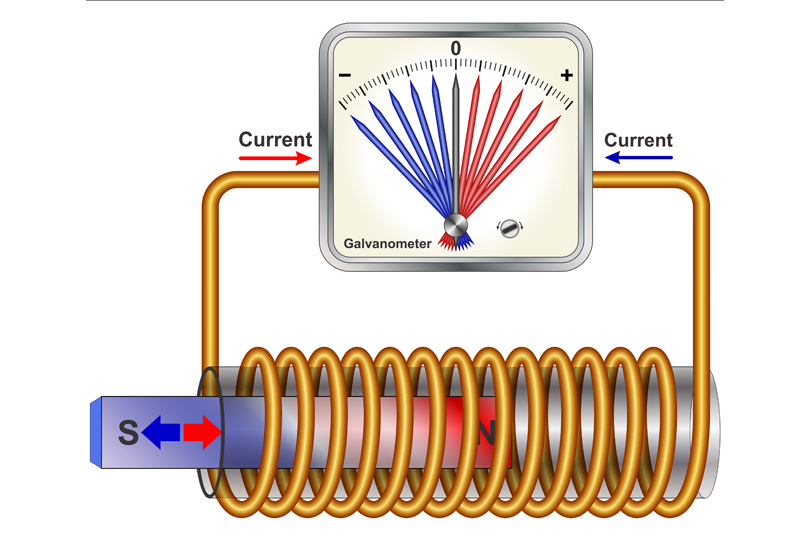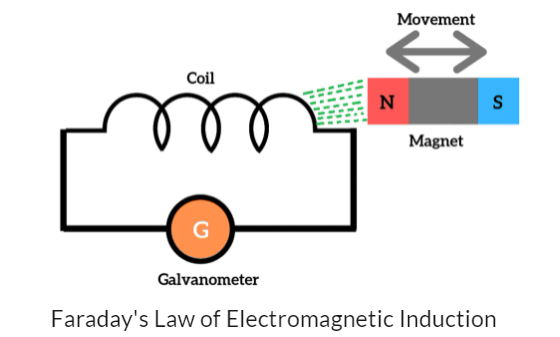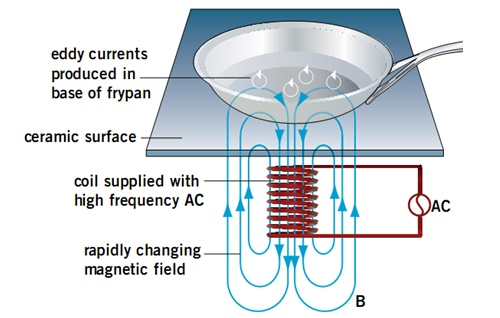Jessica

# Faraday's Law Study Guide

Faraday’s laws of induction are divided into two laws. The first one explains the generation of EMF, and the second one explains the magnitude of the EMF.

# INTRODUCTION

We’re all familiar with gas stoves and electric skillets. These devices have a source of heat over which a frying pan or a vessel is placed. About a decade ago, induction hobs or stoves exploded into the market that could perform the same heating as a conventional stove but without a visible source of heat. What if we told you that these slick devices have more in common with the electrical transformer on your street than they do with cooking tops!? So how is the one used for cooking and the other in power grids? Let’s find out!Source

## FARADAY'S LAW OF INDUCTION

Michael Faraday made some cool discoveries when experimenting with conductors and magnets in 1830. Subsequently, he proposed the laws of electromagnetic induction, which explained the phenomenon of how changing magnetic flux induces a current in a conductor. Faraday’s laws of induction are divided into two laws. The first one explains how an electromotive force (EMF) is generated in a conductor, and the second law quantifies the EMF.

## WHAT DOES FARADAY'S LAW STATE?

According to Faraday’s first law, an EMF is induced in a conductor placed in a changing magnetic field. This phenomenon is called electromagnetic induction. It’s important to remember that electromagnetic induction only occurs when the magnetic field moves or changes in strength. In a closed circuit, the EMF acts like a battery and creates a current that can do work.Source

Consider the setup in the figure above. Every time the magnet is moved in and out of the coil, the galvanometer shows an EMF. The same principle applies to electric generators that use rotating magnetic fields to produce power.

According to the second faraday’s law of electromagnetic induction, the magnitude of the induced EMF in the conductor is directly proportional to the rate of change of magnetic field. Considering the above example, the faster the magnet moves, the more EMF it generates.

Mathematically it can be expressed as:

``````               ε = - N ΔΦ/Δt
``````

ε is the magnitude of the induced EMF N is the number of turns on the coil ΔΦ is the change in magnetic flux Δt is the change in time

The EMF has a negative sign because the induced current flows in a direction to oppose the changing magnetic field, as explained by Lenz’s Law.

## APPLICATION OF FARADAY’S LAWSource

Going back to the induction stove in the introduction, the device produces heat by inducing a current in the cooking vessel. It uses rapidly alternating magnetic flux to generate eddy currents that heat the vessel because of electrical resistance. An electrical transformer alternating current in the primary coil produces a changing magnetic field in the secondary coil. This induces an electrical current in the secondary coil; however, the purpose of a transformer is to increase or decrease voltage.

# CONCLUSION:

• According to Faraday’s first law, an EMF is induced in a conductor placed in a changing magnetic field.
• According to the second faraday’s law of electromagnetic induction, the magnitude of the induced EMF in the conductor is directly proportional to the rate of change of magnetic field.

### FAQs:

1. What is Faraday's law?

Faraday's law physics describes electromagnetic induction in a conductor placed inside a changing magnetic field.

2. What are Faraday's 1st and 2nd laws?

• According to Faraday’s first law, an EMF is induced in a conductor placed in a changing magnetic field.
• According to the second faraday’s law of electromagnetic induction, the magnitude of the induced EMF in the conductor is directly proportional to the rate of change of magnetic field.

We hope you enjoyed studying this lesson and learned something cool about Faraday’s Law! Join our Discord community to get any questions you may have answered and to engage with other students just like you! We promise, it makes studying much more fun! 😎

## SOURCES:

1. 22.2 Discovery of Electromagnetism. https://flexbooks.ck12.org/cbook/ck-12-middle-school-physical-science-flexbook-2.0/section/22.2/primary/lesson/discovery-of-electromagnetism-ms-ps/. Accessed 28 Jan 2022.
2. Faraday’s law of electromagnetic induction. https://www.electricalclassroom.com/faradays-law-of-induction/. Accessed 28 Jan 2022.
3. What Is Faraday’s Law of Induction?. https://www.livescience.com/53509-faradays-law-induction.html. Accessed 28 Jan 2022.
4. Faraday’s Laws of Electromagnetic Induction. https://byjus.com/physics/faradays-law/. Accessed 28 Jan 2022.